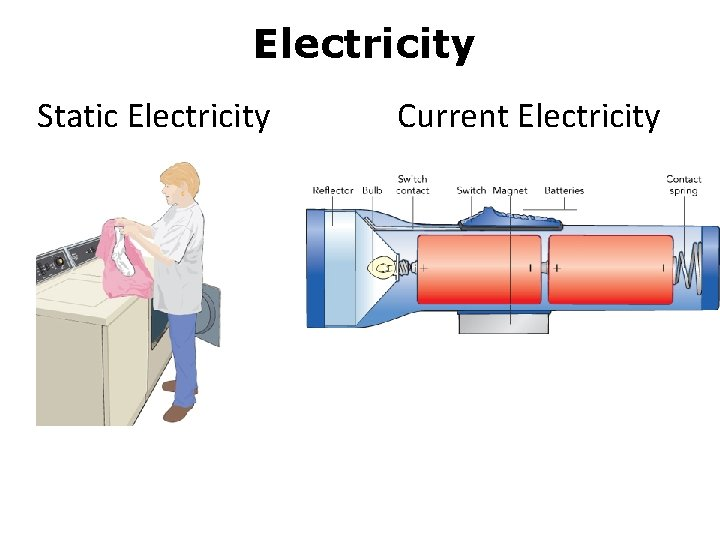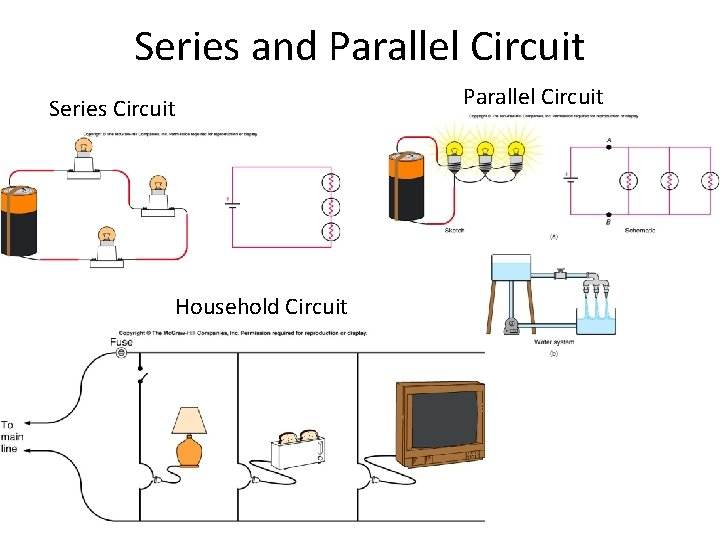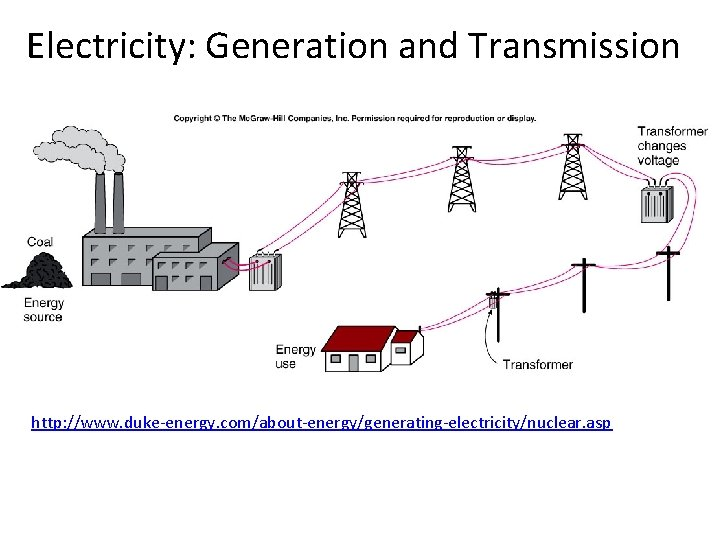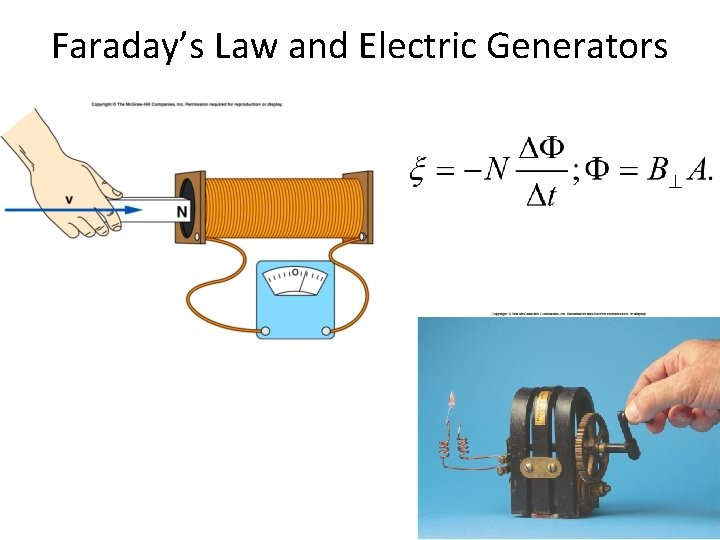Electricity Static Electricity Current Electricity Atomic Particle Charge

• Slides: 12Electricity Static Electricity Current ElectricityAtomic Particle Charge Mass Electron – 1. 6 10 -19 C 9. 11 10 -31 Kg Proton +1. 6 10 -19 C 1. 673 10 -27 Kg Neutron 0 1. 675 10 -27 KgElectric Current The electric current is the amount of charge per unit time that passes through a surface that is perpendicular to the motion of the charges. The SI unit of electric current is the ampere (A), after the French mathematician André Ampére (1775 -1836). 1 A = 1 C/s. Ampere is a large unit for current. In practice milliampere (m. A) and microampere (μA) are used.Electrical Quantities and their Units Quantity Symbol Unit Name Unit Abbreviation Current I ampere A Voltage V volt V Resistance R ohm Ω Power P watt WEmf’s or Voltages of Common Batteries • Car battery = 12 V • AAA, C, D = 1. 5 V • 9 -volt battery = 9 V • Lantern battery = 6 VOhm’s Law Georg Simon Ohm (1787 -1854), a German physicist, discovered Ohm’s law in 1826. This is an experimental law, valid for both alternating current (ac) and direct current (dc) circuits. When you pass an electric current (I) through a resistance (R) there will be a potential difference or voltage (V) created across the resistance. Ohm’s law gives a relationship between the voltage (V), current (I), and resistance (R) as follows: V=IR P 1: An automobile has a 18 -W reading lamp in the ceiling. This lamp operates with a voltage drop of 12 V across it. How much current flows through the lamp? P 2: How much current will flow through a 60 -W household table lamp, when connected to a 120 -volt outlet?Electric Power, P The SI unit of power is watt, after James Watt (1736 -1819), who developed steam engines. Utility companies use the unit kilowatt-hour to measure the electrical energy used by customers. One kilowatt-hour, k. Wh is the energy consumed for one hour at a power rate of 1 k. W.Killowatt-hour (k. Wh) The SI unit of power is watt, after James Watt (1736 -1819), who developed steam engines. Utility companies use the unit kilowatt-hour to measure the electrical energy used by customers. One kilowatt-hour, k. Wh is the energy consumed for one hour at a power rate of 1 k. W. P: An appliance with a power rating of 1500 -W is used 10 hours a day. If the cost of electricity is 12 cents per kilowatt‑hour, how much does it cost to operate this appliance for the month of April?Series and Parallel Circuit Series Circuit Household Circuit Parallel CircuitElectricity: Generation and Transmission http: //www. duke-energy. com/about-energy/generating-electricity/nuclear. aspTransformers A transformer is a device for increasing or decreasing an ac voltage. A transformer consists of a primary coil and a secondary coil, both wound on an iron core. The changing magnetic flux produced by the current in the primary coil induces an emf in the secondary coil. At the far right is the symbol for a transformer.